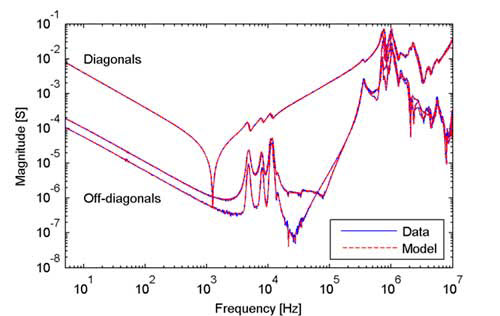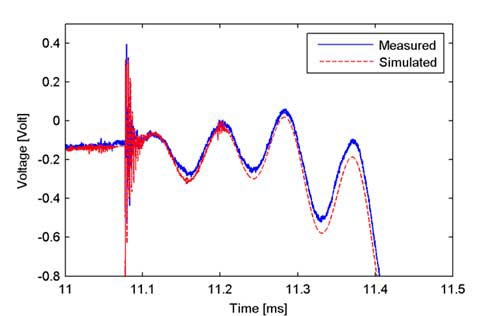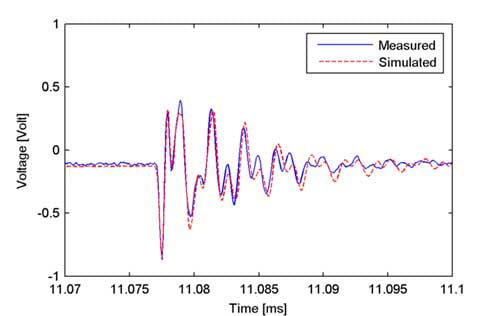Norsk English

# Vector Fitting

Vector Fitting is a robust numerical method for rational approximation in the frequency domain using poles and residues. It permits to calculate multi-port models directly from measured or computed frequency responses. The resulting approximation has guaranteed stable poles that are real or come in complex conjugate pairs, and the model can be directly converted into a state-space model.

## Contact personMeasurement of reactor admittance matrix. Foto: SINTEF/Oddgeir Rokseth

Vector Fitting is widely applied in many engineering societies, from high-voltage power systems to microwave systems and high-speed electronics. The application is typically to calculate a reduced-order passive macromodel from characterizing terminal frequency responses, also known as black-box modeling. Such macromodels permit efficient time domains simulation in circuit simulators such as EMTP and SPICE, using an equivalent circuit or recursive convolution.

This web site gives information about Vector Fitting and provides downloadable open-source Matlab implementations. For instance, download package "Matrix Fitting Toolbox" contains a complete procedure for multi-port rational modeling from frequency domain data, applicable to both admittance (Y-) and scattering (S-) parameter data sets. Rational fitting and passivity enforcement is achieved by two subsequent function calls. The first function call calculates a rational approximation using a fast implementation of Vector Fitting while the second function call enforces passivity of the model by perturbation of residue matrices, thereby guaranteeing the stability of the model when used in time domain simulations. A routine is available for generating netlist for ATP-EMTP, for subsequent time domain simulation. A separate package is also provided which demonstrates time domain simulation using recursive convolution, for models defining admittance, impedance, or scattering parameters.Frequency responses (admittance). Measurements and model extracted from the frequency responses using Matrix Fitting toolbox.Verfiying model accduracy in the time domain. Simulated and measured response, with two alternative time scales.

The Vector Fitting Website